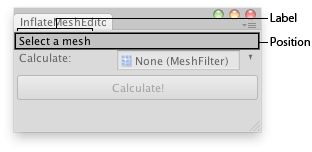﻿ Unity - スクリプティング API: EditorGUI.PrefixLabel

• C#
• JS
• Boo

## スクリプト言語

お好みのスクリプト言語を選択すると、サンプルコードがその言語で表示されます。

# EditorGUI.PrefixLabel

Suggest a change

## Success!

Thank you for helping us improve the quality of Unity Documentation. Although we cannot accept all submissions, we do read each suggested change from our users and will make updates where applicable.

Close

## Sumbission failed

For some reason your suggested change could not be submitted. Please try again in a few minutes. And thank you for taking the time to help us improve the quality of Unity Documentation.

Close

Cancel

public static function PrefixLabel(totalPosition: Rect, id: int, label: GUIContent): Rect;
public static Rect PrefixLabel(Rect totalPosition, int id, GUIContent label);
public static def PrefixLabel(totalPosition as Rect, id as int, label as GUIContent) as Rect

## Parameters

 totalPosition Rectangle on the screen to use in total for both the label and the control. id The unique ID of the control. If none specified, the ID of the following control is used. label Label to show in front of the control.

## Returns

Rect Rectangle on the screen to use just for the control itself.

## Description

Make a label in front of some control.Prefix Label in an Editor Window. Note that most editor controls already have built-in optional labels that can be specified as one of the parameters. PrefixLabel can be used when there is no such built-in label available, or when you're creating your own editor control from scratch. PrefixLabel takes a rect that's the rect for the entire control, including the label, and returns a rect that's for just the control itself, without the label. PrefixLabel also ensures that when the label is clicked, the linked control will get keyboard focus (if the control supports keyboard focus). The ID of the linked control can optionally be specified, or if no ID is given, the label is automatically linked to the following control coming after it.

```	// Inflates a mesh
//
// Usage: Select a mesh and drag it to the object field.
// Press calculate and after finishing just press play and see your mesh growing.
//
// Note: To control the ratio of inflation just change the increaseRatio
// var in the "InflateMesh.js" sript

class InflateMeshEditor extends EditorWindow {

var object : MeshFilter;

static function Init () {
var window = GetWindow (InflateMeshEditor);
window.Show ();
}

function OnGUI () {
var rect = EditorGUILayout.GetControlRect ();
rect = EditorGUI.PrefixLabel (rect, GUIContent ("Select a mesh"));
object = EditorGUI.ObjectField (rect,
"Calculate:",
object,
MeshFilter);

EditorGUI.BeginDisabledGroup (!object);
if(GUI.Button (EditorGUILayout.GetControlRect (), "Calculate!"))
Calculate ();
EditorGUI.EndDisabledGroup ();
}

function Calculate () {
var finalNormals = new Vector3;
var mesh = object.sharedMesh;
var vertices = mesh.vertices;
var normals = mesh.normals;

// Find identical vertices
// this will hold an ID for each vertex, vertices at
// the same position will share the same ID!
var vertexIDs = new int[vertices.length];
var counter : int = 0;

for (var i = 0; i < vertices.length; i++) {
for (var j = 0; j < vertices.length; j++) {
if (vertexIDs[i] == 0) {
counter++;
vertexIDs[i] = counter;
}
if (i != j)
if (vertices[i] == vertices[j] && vertices[i] != 0)
vertexIDs[j] = vertexIDs[i];
}
}

finalNormals = normals;
calculated = 0.5;
// Calcualte average normals
// counter is the highest vertexID, now go through all the groups and collect normal data
for (var k = 1; k <= counter; k++) {
var curAvgNormal : Vector3 = Vector3.zero;
for (var l = 0; l < vertexIDs.length; l++)
if (vertexIDs[l] == k) {
// Add up all the normals of the vertices with identical positions
curAvgNormal += normals[l];
}
curAvgNormal.Normalize(); //Normalize the result
for (var m = 0; m < vertexIDs.length; m++)
if (vertexIDs[m] == k)
finalNormals[m] = curAvgNormal;
}
Debug.Log ("Done Adding Component, press play and see your mesh being inflated!");
}
}
```

And the script attached to the editor script:

```	// InflateMesh.js
private var mesh : Mesh;
private var vertices = new Vector3;
private var normals = new Vector3;
var fNormals = new Vector3;
var increaseRatio = 0.005;
function Start () {
mesh = GetComponent (MeshFilter).mesh;
vertices = mesh.vertices;
normals = mesh.normals;
}

function Update () {
for (var i = 0; i < vertices.length; i++) {
vertices[i] += fNormals[i]  * Time.deltaTime * increaseRatio;
}
mesh.vertices = vertices;
}
```